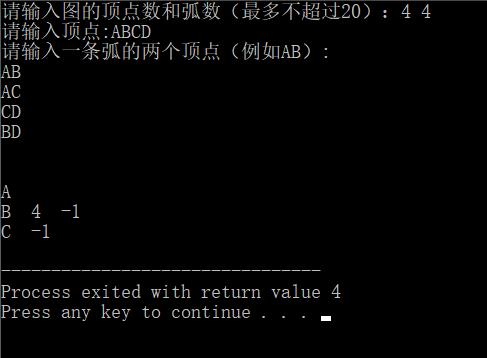【数据结构】用邻接表存储图的简单问题
``````#include<stdio.h>
#define MAX_VERTEX_NUM 20//最多顶点个数
#define ERROR -1

typedef char VertexData;
//边表节点类型定义
typedef struct ArcNode{
struct ArcNode *nextarc;//指向下一条弧的指针
}ArcNode;
//表头节点类型定义
typedef struct VertexNode{
VertexData data;
ArcNode *firstarc;//指向该顶点第一条弧的指针；
int order;
}VertexNode;
typedef struct{
VertexNode vertex[MAX_VERTEX_NUM];
int vexnum,arcnum;

{
int j=ERROR,k;
for(k=0;k<G->vexnum;k++)
{
if(G->vertex[k].data==v)
{
j=G->vertex[k].order;
break;
}
}
return(j);
}

{
printf("请输入图的顶点数和弧数（最多不超过20）：");
scanf("%d %d",&G->vexnum,&G->arcnum);
printf("请输入顶点:");
int i,j,k;
char v1,v2;
for(i=0;i<G->vexnum;i++)
{
scanf("%c",&(G->vertex[i].data));
G->vertex[i].order=i+1;
G->vertex[i].firstarc=NULL;

}
printf("请输入一条弧的两个顶点（例如AB）:\n");
for(k=0;k<G->vexnum;k++)
{
scanf("%c %c",&v1,&v2);
ArcNode *p,*q;
p=(ArcNode*)malloc(sizeof(ArcNode));
q=(ArcNode*)malloc(sizeof(ArcNode));

i=LocateVertex(G,v1);
j=LocateVertex(G,v2);
p->nextarc=G->vertex[i].firstarc;
q->nextarc=G->vertex[j].firstarc;
G->vertex[i].firstarc=p;
G->vertex[j].firstarc=q;
fflush(stdin);
}

}
{
int i,j;
for(i=0;i<G->vexnum;i++)
{
printf("%c  ",G->vertex[i].data);
ArcNode *p;
p=G->vertex[i].firstarc;
while(p!=NULL)
{
p=p->nextarc;
}
printf("\n");

}
}

void main()
{
CreateGraph(&G);
print(&G);
}
``````1个回答#define MaxVertexNum 20 /* 最大顶点数设为20 */ #define INFINITY 32767 /* ∞设为双字节无符号整数的最大值32767*/ typedef char VertexType; /* 顶点类型设为字符型 */ typedef int EdgeType; /* 边的权值设为整型 */ enum GraphType { DG, UG, DN, UN }; /* 有向图,无向图,有向网图,无向网图*/ typedef struct { VertexType Vertices[ MaxVertexNum ]; /* 顶点表 */ EdgeType Edges[ MaxVertexNum ］[ MaxVertexNum ]; /* 邻接矩阵，即边表 */ int n, e; /* 顶点数n和边数e */ enum GraphType GType; /* 图的类型分4种：UG、DG、UN、DN */ } MGraph; /* MGragh是以邻接矩阵存储的图类型 */ void CreateMGraph ( MGraph *G ) { int i, j, k, w; G-> GType = UN; /* Undirected Network 无向网图 */ printf( "请输入顶点数和边数(输入格式为:顶点数, 边数):\n" ); scanf( "%d, %d",&(G->n), &(G->e) ); /* 输入顶点数和边数 */ printf("请输入顶点信息(输入格式为:顶点号<CR>):\n"); for ( i = 0; i < G->n; i++ ) scanf( "%c",&(G-> Vertices[i]) ); /* 输入顶点信息，建立顶点表 */ for ( i = 0; i < G->n; i++ ) for ( j = 0; j < G->n; j++ ) G->Edges[i][j] = INFINITY; /* 初始化邻接矩阵 */ printf( "请输入每条边对应的两个顶点的序号和权值，输入格式为:i, j, w:\n" ); for ( k = 0; k < G->e; k++ ) { scanf("%d,%d,%d ",&i, &j, &w); /* 输入e条边上的权，建立邻接矩阵 */ G->Edges[i][j] = w; G->Edges[j][i] = w; /* 因为无向网图的邻接矩阵是对称的 */ } }![图片说明](https://img-ask.csdn.net/upload/201611/20/1479642113_25182.png)

c++数据结构有向网相关问题

1.建立邻接表存储的图； 2.进行拓扑排序； 3.输出拓扑排序序列。 求完整过程

``` /* 树转换为二叉树 题目说明：建立一棵树，将其转化为二叉树，并给出该二叉树的先序遍历序列。 要求：树为任意输入，以孩子链表法存储，转换所得二叉树以二叉链表为存储结构。 */ #include <stdio.h> #include <stdlib.h> #define MAX_TREE_SIZE 100 #define OVERFLOW -2 #define TRUE 1 #define FALSE 0 typedef int TElemType; typedef int Status; #define OK 1 void visit(TElemType e) { printf("%d", e); } typedef struct CTNode { //孩子结点 int child; struct CTNode *next; }*ChildPtr; typedef struct { TElemType data;//结点值 ChildPtr firstchild; //孩子链表头指针 //可增设一个双亲的域，能方便地找到其双亲。int Parent; }CTBox; typedef struct { CTBox nodes[MAX_TREE_SIZE]; //建立顺序表头结构 int n, r; //结点数和根的位置 }CTree; typedef struct BTNode { int data; CTBox list[MAX_TREE_SIZE]; struct BTNode *lchild; struct BTNode *rchild;//兄弟结点 }BTNode; typedef struct { CTBox list[MAX_TREE_SIZE];//邻接表表头 int n;//结点个数 }Tree;//定义树 //--------------树的孩子链表存储表示------------------- Status CreateTree(CTree &T) { //构建一棵树 int i; printf("请输入结点数及根结点的位置下标：\n"); scanf("%d %d",&T.n, &T.r); printf("请输入各节点的值\n"); for(i = 0; i < T.n; ++i) { scanf("%d",&T.nodes[i].data); T.nodes[i].firstchild = NULL; } printf("创建每个结点的孩子结点...\n"); system("pause"); for(i =0; i < T.n; ++i) { printf("请输入位置为%d的结点的孩子个数（>=0）（有孩子则输入孩子们的位置，无则输入0）：\n",i); int nChild=0; scanf("%d",&nChild); ChildPtr p=NULL; //p指向插入孩子位置的前一个位置 ChildPtr q=NULL; //q用于提示即将插入的新b孩子链表结点 for(int j = 0; j < nChild; ++j) { q= (ChildPtr)malloc(sizeof(struct CTNode));//为孩子的位置开辟一个空间 if(!q) exit(OVERFLOW); scanf("%d",&(q->child)); //将孩子链表结点置入位置值 q->next = NULL; if(j == 0)//将孩子链表头结点指向孩子链表第一个结点 { T.nodes[i].firstchild = q; } else { p->next = q; } p = q; } } return OK; } Status PrintChild(const ChildPtr &C) {//打印出结点的孩子 if(C) { ChildPtr p; p = C; while(p) { printf("[%d| ] ->",p->child); p = p->next; } printf("^"); //return TRUE; } else { printf("^"); //return FALSE; } } void PrintChildTree(const CTree &T)//输出树的结点及他们的孩子 { printf("\n位置%d为树的根%d\n\n", T.r, T.nodes[T.r].data); for(int i = 0; i < T.n; ++i) { printf("位置%d,结点值[%d| ] ->", i, T.nodes[i].data); PrintChild(T.nodes[i].firstchild); printf("\n"); } printf("---建立树成功----"); } //--------------树转化为二叉树-------------- ？ void treeToBtree(Tree *t,BTNode *bt,int i) {//树转二叉树 if(t != NULL) { CTBox *p = &(t->list[i]); bt->list[i]= t->list[i];//二叉树的头结点等于树的头结点 ChildPtr b = p->firstchild;//该结点的孩子结点 BTNode *q = bt->lchild;//二叉树的左孩子结点 while(b)//把该层的树全部转为二叉树 { treeToBtree(t,q,b->child);//若该结点以及该结点的子孙结点转为二叉树 b = b->next;//指向树结点下一个孩子结点 q = q->rchild;//指向二叉树的右孩子结点 } } } void PrintAsTree(BTNode *T,int i) //显示转化结果 { int cnt; if (T!=NULL) { printf("转化后的二叉树："); for (cnt=1; cnt<i; cnt++) //visit(T->data); printf("\n"); printf("位置%d,结点值[%d| ] ->", cnt, T->data); PrintAsTree(T->lchild, i+1); //遍历左孩子 PrintAsTree(T->rchild, i); //遍历右孩子 } } //---------------二叉树的先序遍历---------------- void PreOrder(BTNode *T) { if(T==NULL) return; else { printf("二叉树的先序遍历结果为："); printf("%d--",T->data); PreOrder(T->lchild); PreOrder(T->rchild); } } int main() { CTree T; Tree *Y; BTNode *bt; ChildPtr C; int i; i=T.n; CreateTree(T); PrintChildTree(T);//输出普通树的结点值 PrintChild(C); //输出各结点的孩子结点值 treeToBtree(Y,bt,i);//树转化为二叉树 PrintAsTree(bt,i);//输出转化后的二叉树 PreOrder(bt); //输出二叉树的先序遍历序列 return 0; } ```

《奇巧淫技》系列-python！！每天早上八点自动发送天气预报邮件到QQ邮箱

11月8日，由中国信息通信研究院、中国通信标准化协会、中国互联网协会、可信区块链推进计划联合主办，科技行者协办的2019可信区块链峰会将在北京悠唐皇冠假日酒店开幕。 　　区块链技术被认为是继蒸汽机、电力、互联网之后，下一代颠覆性的核心技术。如果说蒸汽机释放了人类的生产力，电力解决了人类基本的生活需求，互联网彻底改变了信息传递的方式，区块链作为构造信任的技术有重要的价值。 　　1...

C语言魔塔游戏

Android性能优化(4)：UI渲染机制以及优化

Python爬虫爬取淘宝，京东商品信息

Java工作4年来应聘要16K最后没要,细节如下。。。

SpringBoot2.x系列教程（三十六）SpringBoot之Tomcat配置
Spring Boot默认内嵌的Tomcat为Servlet容器，关于Tomcat的所有属性都在ServerProperties配置类中。同时，也可以实现一些接口来自定义内嵌Servlet容器和内嵌Tomcat等的配置。 关于此配置，网络上有大量的资料，但都是基于SpringBoot1.5.x版本，并不适合当前最新版本。本文将带大家了解一下最新版本的使用。 ServerProperties的部分源...
Python绘图，圣诞树，花，爱心 | Turtle篇

CPU对每个程序员来说，是个既熟悉又陌生的东西？ 如果你只知道CPU是中央处理器的话，那可能对你并没有什么用，那么作为程序员的我们，必须要搞懂的就是CPU这家伙是如何运行的，尤其要搞懂它里面的寄存器是怎么一回事，因为这将让你从底层明白程序的运行机制。 随我一起，来好好认识下CPU这货吧 把CPU掰开来看 对于CPU来说，我们首先就要搞明白它是怎么回事，也就是它的内部构造，当然，CPU那么牛的一个东...

2020年全新Java学习路线图，含配套视频，学完即为中级Java程序员！！

B 站上有哪些很好的学习资源?

Web播放器解决了在手机浏览器和PC浏览器上播放音视频数据的问题，让视音频内容可以不依赖用户安装App，就能进行播放以及在社交平台进行传播。在视频业务大数据平台中，播放数据的统计分析非常重要，所以Web播放器在使用过程中，需要对其内部的数据进行收集并上报至服务端，此时，就需要对发生在其内部的一些播放行为进行事件监听。 那么Web播放器事件监听是怎么实现的呢？ 01 监听事件明细表 名...
3万字总结，Mysql优化之精髓

HTML5适合的情人节礼物有纪念日期功能

Python新型冠状病毒疫情数据自动爬取+统计+发送报告+数据屏幕（三）发送篇